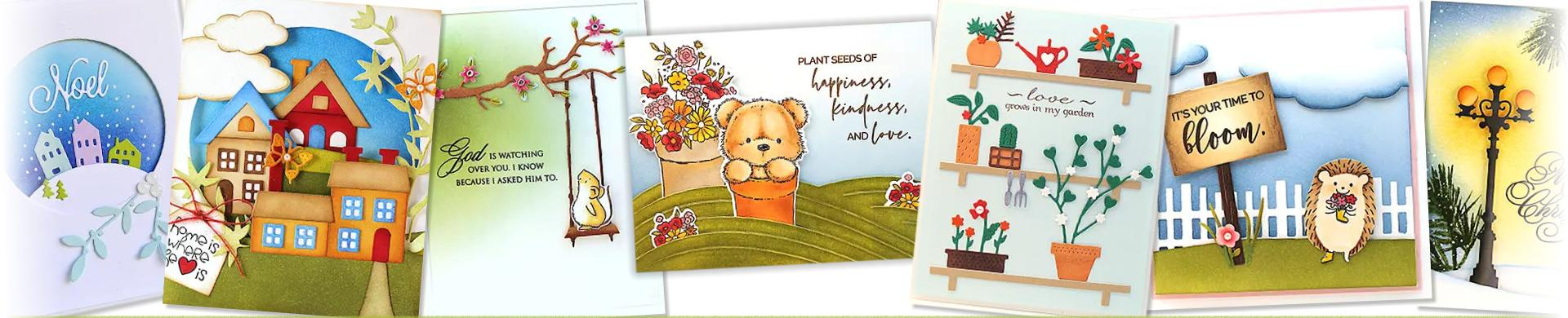# Penny Black Cutting Dies - Scene BuildersDie cut elements to make background scenes on your cards with these handy dies from Penny Black.
• Brand
1. \$11.92
2. \$19.88
3. \$8.94
4. \$23.85
5. \$17.88
6. \$11.92
7. \$9.93
8. \$10.92
9. \$12.92
10. \$14.90
11. \$9.93
12. \$9.93
13. \$17.88
14. \$11.92
15. \$13.91
16. \$12.92
17. \$11.92
18. \$15.90
19. \$15.90
20. \$12.92
21. \$17.88
22. \$15.90
23. \$12.92
24. \$18.88
25. \$19.88
26. \$12.92
27. \$10.92
28. \$12.92
29. \$19.88
30. \$15.90
31. \$19.88
32. \$12.92
33. \$10.92
34. \$17.88
35. \$14.90
36. \$15.90
37. \$14.90
38. \$10.92
39. \$19.88
40. \$14.90
41. \$14.90
42. \$24.85
43. \$21.87
44. \$19.88
45. \$14.41
46. \$10.92
47. \$18.88
48. \$11.92
49. \$13.91
50. \$16.89
51. \$8.44